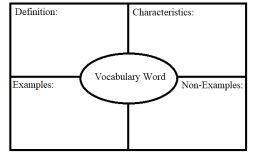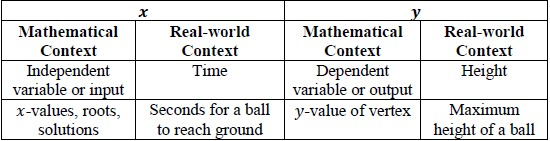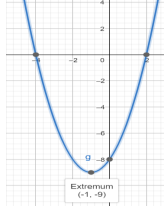# MA.912.AR.3.6Export Print
Given an expression or equation representing a quadratic function, determine the vertex and zeros and interpret them in terms of a real-world context.
General Information
Subject Area: Mathematics (B.E.S.T.)
Strand: Algebraic Reasoning
Status: State Board Approved

## Benchmark Instructional Guide

### Terms from the K-12 Glossary

• $x$-intercept
• $y$-intercept

### Vertical Alignment

Previous Benchmarks

Next Benchmarks

### Purpose and Instructional Strategies

In grade 8, students multiplied two linear expressions to obtain a quadratic expression. In Algebra I, students transform a quadratic function to highlight and interpret its vertex or its zeroes. In later courses, students will determine key features of higher degree polynomials.
• Instruction includes making connections to various forms of quadratic equations to show their equivalency. Students should understand and interpret when one form might be more useful than other depending on the context.
• Standard Form
Can be described by the equation $y$ = $a$$x$2+ $b$$x$ + $c$, where $a$, $b$ and $c$ are any rational number. This form can be useful when identifying the $y$-intercept.
• Factored form
Can be described by the equation $y$ = $a$($x$$r$1)($x$$r$2), where $r$1 and  $r$2 are real numbers and the roots, or $x$-intercepts. This form can be useful when identifying the $x$-intercepts, or roots.
• Vertex form
Can be described by the equation $y$ = $a$($x$$h$)2 + $k$, where the point ($h$, $k$) is the vertex. This form can be useful when identifying the vertex.
• Instruction includes the use of $x$-$y$ notation and function notation.
• Most contexts for this benchmark will present functions in standard form. Depending on their perspectives, students might take several approaches to determine vertices and zeros. Have students discuss strategies they might use. Let students know they should use the approach that is most efficient for them (MTR.3.1).
• To determine zeros, students could use the quadratic formula or convert the function into factored form, or complete the square, or use Loh’s method.
• To determine the vertex, students could convert the function into vertex form or determine the axis of symmetry ($x$ = $\frac{\text{−b}}{\text{2a}}$). Calculate this value from one of the previous  functions discussed and guide students to see that the vertex of each parabola falls on the line of symmetry. Considering this, they can substitute that $y$-value into the function to determine the corresponding $y$-value of the vertex.

### Common Misconceptions or Errors

• Some students may have difficulty interpreting the meaning of the zeros and vertex.

### Strategies to Support Tiered Instruction

• Teacher provides a graphic organizer for key terms (zeros and vertex) that can be created using information provided in a given problem.• Teacher co-creates a graphic organizer to compare real-world and mathematical contexts related to quadratics.
• For example, the chart below can be used to compare mathematical terms with real-world context.• Teacher provides a visual aid, including using graphing software, to interpret the zeros and vertex of a quadratic function. Students can compare definitions and examples to determine the zeros and vertex of the parabola.
• For example, the roots of the graph shown are (2, 0) and (−4, 0). The locations on the graph that intersect the $x$-axis are the roots. The roots are also the solutions of the quadratic. The vertex of the graph shown is (−1, −9). The vertex is the minimum or maximum point (sometimes called the extrema) of the parabola. The vertex is also the point where the quadratic changes from decreasing to increasing or from increasing to decreasing, and is halfway between the roots.Instructional Task 1 (MTR.3.1, MTR.6.1, MTR.7.1
• A diver jumps off a cliff 5 meters high into a lake. The diver’s position can be represented by the function $h$($t$) = −4.9$t$2+ 1.5$t$ + 5, where $h$ represents the diver’s height relative to the lake’s surface and $t$ represents the time in seconds.
• Part A. Determine the roots of the function described.
• Part B. Interpret each with respect to the situation.

• A local campground charges \$23.50 per night per campsite. They average about 32 campsites rented each night. A recent survey indicated that for every \$0.50 decrease, the number of campsites rented increases by five.
• Part A. Write a quadratic equation that describes this situation.
• Part B. Determine the vertex and zeroes of the function described.
• Part C. Interpret each with respect to the situation.
• Part D. What price will maximize revenue?

### Instructional Items

Instructional Item 1
• Marcus just purchased a Super Bouncy Ball from a local toy store. Once outside, he throws it down to see how high the ball will bounce. The function $h$($x$) = 2$x$2   + 24$x$ − 31.5 represents the height, $h$ in inches, above the top of his head of the ball as it relates to its horizontal distance from Marcus $x$, in inches. Find the zeros and vertex of this function and interpret the meaning of each.

*The strategies, tasks and items included in the B1G-M are examples and should not be considered comprehensive.

## Related Courses

This benchmark is part of these courses.
1200310: Algebra 1 (Specifically in versions: 2014 - 2015, 2015 - 2022, 2022 and beyond (current))
1200320: Algebra 1 Honors (Specifically in versions: 2014 - 2015, 2015 - 2022, 2022 and beyond (current))
1200380: Algebra 1-B (Specifically in versions: 2014 - 2015, 2015 - 2022, 2022 and beyond (current))
1200400: Foundational Skills in Mathematics 9-12 (Specifically in versions: 2014 - 2015, 2015 - 2022, 2022 and beyond (current))
7912090: Access Algebra 1B (Specifically in versions: 2014 - 2015, 2015 - 2018, 2018 - 2019, 2019 - 2022, 2022 and beyond (current))
1200315: Algebra 1 for Credit Recovery (Specifically in versions: 2014 - 2015, 2015 - 2022, 2022 and beyond (current))
1200385: Algebra 1-B for Credit Recovery (Specifically in versions: 2014 - 2015, 2015 - 2022, 2022 and beyond (current))
7912075: Access Algebra 1 (Specifically in versions: 2014 - 2015, 2015 - 2018, 2018 - 2019, 2019 - 2022, 2022 and beyond (current))

## Related Access Points

Alternate version of this benchmark for students with significant cognitive disabilities.
MA.912.AR.3.AP.6: Given an expression or equation representing a quadratic function in vertex form, determine the vertex and zeros.

## Related Resources

Vetted resources educators can use to teach the concepts and skills in this benchmark.

## Formative Assessments

Students are asked to compare two quadratic functions, one given by a table and the other by a function.

Type: Formative Assessment

Students are asked to identify the zeros of polynomials, without the use of technology, and then describe what the zeros of a polynomial indicate about its graph.

Type: Formative Assessment

A Home for Fido:

Students are asked to rewrite a quadratic function in an equivalent form by completing the square and to use this form to identify the vertex of the graph and explain its meaning in context.

Type: Formative Assessment

Launch From a Hill:

Students are asked to factor and find the zeros of a polynomial function given in context.

Type: Formative Assessment

## Original Student Tutorials

Highs and Lows Part 2: Completing the Square:

Learn the process of completing the square of a quadratic function to find the maximum or minimum to discover how high a dolphin jumped in this interactive tutorial.

This is part 2 of a 2 part series. Click HERE to open part 1.

Type: Original Student Tutorial

Highs and Lows Part 1: Completing the Square:

Learn the process of completing the square of a quadratic function to find the maximum or minimum to discover how high a dolphin jumped in this interactive tutorial.

This is part 1 of a 2 part series. Click HERE to open Part 2.

Type: Original Student Tutorial

Finding the Zeros of Quadratic Functions:

Learn to find the zeros of a quadratic function and interpret their meaning in real-world contexts with this interactive tutorial.

Type: Original Student Tutorial

Finding the Maximum or Minimum of a Quadratic Function:

Learn to complete the square of a quadratic expression and identify the maximum or minimum value of the quadratic function it defines. In this interactive tutorial, you'll also interpret the meaning of the maximum and minimum of a quadratic function in a real world context.

Type: Original Student Tutorial

Follow as we discover key features of a quadratic equation written in vertex form in this interactive tutorial.

Type: Original Student Tutorial

## Perspectives Video: Expert

Type: Perspectives Video: Expert

## Tutorials

Graphing Quadractic Functions in Vertex Form:

This tutorial will help the students to identify the vertex of a parabola from the equation, and then graph the parabola.

Type: Tutorial

This tutorial helps the learners to graph the equation of a quadratic function using the coordinates of the vertex of a parabola and its x- intercepts.

Type: Tutorial

## MFAS Formative Assessments

A Home for Fido:

Students are asked to rewrite a quadratic function in an equivalent form by completing the square and to use this form to identify the vertex of the graph and explain its meaning in context.

Students are asked to compare two quadratic functions, one given by a table and the other by a function.

Launch From a Hill:

Students are asked to factor and find the zeros of a polynomial function given in context.

Students are asked to identify the zeros of polynomials, without the use of technology, and then describe what the zeros of a polynomial indicate about its graph.

## Original Student Tutorials Mathematics - Grades 9-12

Finding the Maximum or Minimum of a Quadratic Function:

Learn to complete the square of a quadratic expression and identify the maximum or minimum value of the quadratic function it defines. In this interactive tutorial, you'll also interpret the meaning of the maximum and minimum of a quadratic function in a real world context.

Finding the Zeros of Quadratic Functions:

Learn to find the zeros of a quadratic function and interpret their meaning in real-world contexts with this interactive tutorial.

Follow as we discover key features of a quadratic equation written in vertex form in this interactive tutorial.

Highs and Lows Part 1: Completing the Square:

Learn the process of completing the square of a quadratic function to find the maximum or minimum to discover how high a dolphin jumped in this interactive tutorial.

This is part 1 of a 2 part series. Click HERE to open Part 2.

Highs and Lows Part 2: Completing the Square:

Learn the process of completing the square of a quadratic function to find the maximum or minimum to discover how high a dolphin jumped in this interactive tutorial.

This is part 2 of a 2 part series. Click HERE to open part 1.

## Student Resources

Vetted resources students can use to learn the concepts and skills in this benchmark.

## Original Student Tutorials

Highs and Lows Part 2: Completing the Square:

Learn the process of completing the square of a quadratic function to find the maximum or minimum to discover how high a dolphin jumped in this interactive tutorial.

This is part 2 of a 2 part series. Click HERE to open part 1.

Type: Original Student Tutorial

Highs and Lows Part 1: Completing the Square:

Learn the process of completing the square of a quadratic function to find the maximum or minimum to discover how high a dolphin jumped in this interactive tutorial.

This is part 1 of a 2 part series. Click HERE to open Part 2.

Type: Original Student Tutorial

Finding the Zeros of Quadratic Functions:

Learn to find the zeros of a quadratic function and interpret their meaning in real-world contexts with this interactive tutorial.

Type: Original Student Tutorial

Finding the Maximum or Minimum of a Quadratic Function:

Learn to complete the square of a quadratic expression and identify the maximum or minimum value of the quadratic function it defines. In this interactive tutorial, you'll also interpret the meaning of the maximum and minimum of a quadratic function in a real world context.

Type: Original Student Tutorial

Follow as we discover key features of a quadratic equation written in vertex form in this interactive tutorial.

Type: Original Student Tutorial

## Perspectives Video: Expert

Type: Perspectives Video: Expert

## Tutorials

Graphing Quadractic Functions in Vertex Form:

This tutorial will help the students to identify the vertex of a parabola from the equation, and then graph the parabola.

Type: Tutorial